Share

# HC Verma solutions for Class 12 Concepts of Physics Vol. 2 chapter 7 - Electric Field and Potential [Latest edition]

Textbook page

#### Chapters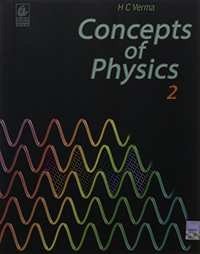## Chapter 7: Electric Field and Potential

#### HC Verma solutions for Class 12 Concepts of Physics Vol. 2 Chapter 7 Electric Field and Potential Exercise Short Answers [Page 119]

Short Answers | Q 1 | Page 119

The charge on a proton is +1.6 × 10−19 C and that on an electron is −1.6 × 10−19 C. Does it mean that the electron has 3.2 × 10−19 C less charge than the proton?

Short Answers | Q 2 | Page 119

Is there any lower limit to the electric force between two particles placed at a separation of 1 cm?

Short Answers | Q 3 | Page 119

Consider two particles A and B of equal charges placed at some distance. Particle A is slightly displaced towards B. Does the force on B increase as soon as particle A is displaced? Does the force on particle A increase as soon as it is displaced?

Short Answers | Q 4 | Page 119

Can a gravitational field be added vectorially to an electric field to get a total field?

Short Answers | Q 5 | Page 119

Why does a phonograph record attract dust particles just after it is cleaned?

Short Answers | Q 6 | Page 119

Does the force on a charge due to another charge depend on the charges present nearby?

Short Answers | Q 7 | Page 119

In some old texts it is mentioned that 4π lines of force originate from each unit positive charge. Comment on the statement in view of the fact that 4π is not an integer.

Short Answers | Q 8 | Page 119

Can two equipotential surfaces cut each other?

Short Answers | Q 9 | Page 119

If a charge is placed at rest in an electric field, will its path be along a line of force? Discuss the situation when the lines of force are straight and when they are curved.

Short Answers | Q 10 | Page 119

Consider the situation shown in the figure. What are the signs of q1 and q2? If the lines are drawn in proportion to the charges, what is the ratio q1/q2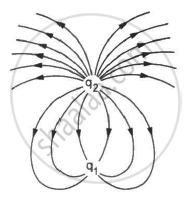Short Answers | Q 11 | Page 119

A point charge is taken from a point A to a point B in an electric field. Does the work done by the electric field depend on the path of the charge?

Short Answers | Q 12 | Page 119

It is said that the separation between the two charges forming an electric dipole should be small. In comparison to what should this separation be small?

Short Answers | Q 13 | Page 119

The number of electrons in an insulator is of the same order as the number of electrons in a conductor. What is then the basic difference between a conductor and an insulator ?

Short Answers | Q 14 | Page 119

When a charged comb is brought near a small piece of paper, it attracts the piece. Does the paper become charged when the comb is brought near it?

#### HC Verma solutions for Class 12 Concepts of Physics Vol. 2 Chapter 7 Electric Field and Potential Exercise Short Answers, MCQ [Pages 119 - 120]

Short Answers | Q 1 | Page 119

Figure shows some of the electric field lines corresponding to an electric field. The figure suggests that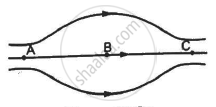•  EA > EB > EC

• EA = EB = EC

•  EA = EC > EB

• EA = EC < EB

MCQ | Q 2 | Page 119

When the separation between two charges is increased, the electric potential energy of the charges

• increases

•  decreases

• remains the same

• may increase or decrease

MCQ | Q 3 | Page 119

If a positive charge is shifted from a low-potential region to a high-potential region, the electric potential energy

• increases

• decreases

•  remains the same

• may increase of decrease

MCQ | Q 4 | Page 120

Two equal positive charges are kept at points A and B. The electric potential at the points between A and B (excluding these points) is situated while moving from A to B. The potential

• continuously increases

•  continuously decreases

•  increases then decreases

• decreases then increases

MCQ | Q 5 | Page 120

The electric field at the origin is along the positive x-axis. A small circle is drawn with the centre at the origin, cutting the axes at points A, B, C and D with coordinates (a, 0), (0, a), (−a, 0), (0, −a), respectively. Out of the points on the periphery of the circle, the potential is minimum at

• A

• B

• C

• D

MCQ | Q 6 | Page 120

If a body is charged by rubbing it, its weight

• remains precisely constant

•  increases slightly

• decreases slightly

• may increase slightly or may decrease slightly

MCQ | Q 7 | Page 120

An electric dipole is placed in a uniform electric field. The net electric force on the dipole

• is always zero

•  depends on the orientation of the dipole

•  can never be zero

•  depends on the strength of the dipole

MCQ | Q 8 | Page 120

Consider the situation in the figure. The work done in taking a point charge from P to Ais WA, from P to B is WB and from P to C is WC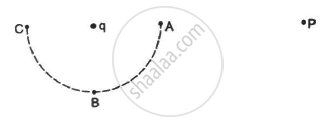• WA < WB < W

• WA > WB > W

• WA = WB = W

• None of these

MCQ | Q 9 | Page 120

A point charge q is rotated along a circle in an electric field generated by another point charge Q. The work done by the electric field on the rotating charge in one complete revolution is

• zero

•  positive

•  negative

• zero if the charge Q is at the centre, otherwise non-zero

#### HC Verma solutions for Class 12 Concepts of Physics Vol. 2 Chapter 7 Electric Field and Potential Exercise MCQ [Page 120]

MCQ | Q 1 | Page 120

Mark out the correct options.

•  The total charge of the universe is constant.

• The total positive charge of the universe is constant.

•  The total negative charge of the universe is constant.

• The total number of charged particles in the universe is constant.

MCQ | Q 2 | Page 120

A point charge is brought inside an electric field. The electric field at a nearby point
(a) will increase if the charge is positive
(b) will decrease if the charge is negative
(c) may increase if the charge is positive
(d) may decrease if the charge is negative

MCQ | Q 3 | Page 120

The electric field and the electric potential at a point are E and V, respectively.

•  If E = 0, V must be zero.

•  If V = 0, E must be zero.

• If E ≠ 0, V cannot be zero.

• If V ≠0, E cannot be zero.

• None of the above.

MCQ | Q 4 | Page 120

Electric potential decreases uniformly from 120 V to 80 V, as one moves on the x-axis from x = −1 cm to x = +1 cm. The electric field at the origin

(a) must be equal to 20 Vcm−1
(b) may be equal to 20 Vcm−1
(c) may be greater than 20 Vcm−1
(d) may be less than 20 Vcm−1

MCQ | Q 5 | Page 120

Which of the following quantities does not depend on the choice of zero potential or zero potential energy?
(a) Potential at a point
(b) Potential difference between two points
(c) Potential energy of a two-charge system
(d) Change in potential energy of a two-charge system

MCQ | Q 6 | Page 120

An electric dipole is placed in an electric field generated by a point charge.

• The net electric force on the dipole must be zero.

•  The net electric force on the dipole may be zero.

•  The torque on the dipole due to the field must be zero.

• The torque on the dipole due to the field may be zero.

MCQ | Q 7 | Page 120

A proton and an electron are placed in a uniform electric field.

• The electric forces acting on them will be equal.

•  The magnitudes of the forces will be equal.

•  Their accelerations will be equal.

• The magnitudes of their accelerations will be equal.

MCQ | Q 8 | Page 120

The electric field in a region is directed outward and is proportional to the distance rfrom the origin. Taking the electric potential at the origin to be zero,

•  it is uniform in the region

• it is proportional to r

•  it is proportional to r

•  it increases as one moves away from the origin

#### HC Verma solutions for Class 12 Concepts of Physics Vol. 2 Chapter 7 Electric Field and Potential [Pages 121 - 124]

Q 1 | Page 121

Find the dimensional formula of ε0.

Q 2 | Page 121

A charge of 1.0 C is placed at the top of your college building and another equal charge at the top of your house. Take the separation between the two charges to be 2.0 km. Find the force exerted by the charges on each other. How many times your weight is this force?

Q 3 | Page 121

At what separation should two equal charges, 1.0 C each, be placed, so that the force between them equals the weight of a 50 kg person?

Q 4 | Page 121

Two equal charges are placed at a separation of 1.0 m. What should be the magnitude of the charges, so that the force between them equals the weight of a 50 kg person?

Q 5 | Page 121

Find the electric force between two protons separated by a distance of 1 fermi (1 fermi = 10−15 m). The protons in a nucleus remain at a separation of this order.

Q 6 | Page 121

Two charges 2.0 × 10−6 C and 1.0 × 10−6 C are placed at a separation of 10 cm. Where should a third charge be placed, such that it experiences no net force due to these charges?

Q 7 | Page 121

Suppose the second charge in the previous problem is −1.0 × 106 C. Locate the position where a third charge will not experience a net force.

Q 8 | Page 121

Two charged particles are placed 1.0 cm apart. What is the minimum possible magnitude of the electric force acting on each charge?

Q 9 | Page 121

Estimate the number of electrons in 100 g of water. How much is the total negative charge on these electrons?

Q 10 | Page 121

Suppose all the electrons of 100 g water are lumped together to form a negatively-charged particle and all the nuclei are lumped together to form a positively-charged particle. If these two particles are placed 10.0 cm away from each other, find the force of attraction between them. Compare it with your weight.

Q 11 | Page 121

Consider a gold nucleus to be a sphere of radius 6.9 fermi in which protons and neutrons are distributed. Find the force of repulsion between two protons situated at largest separation. Why do these protons not fly apart under this repulsion?

Q 12 | Page 121

Two insulating small spheres are rubbed against each other and placed 1 cm apart. If they attract each other with a force of 0.1 N, how many electrons were transferred from one sphere to the other during rubbing?

Q 13 | Page 121

NaCl molecule is bound due to the electric force between the sodium and the chlorine ions when one electron of sodium is transferred to chlorine. Taking the separation between the ions to be 2.75 × 10−8 cm, find the force of attraction between them. State the assumptions (if any) that you have made.

Q 14 | Page 121

Find the ratio of the electrical and gravitational forces between two protons.

Q 15.1 | Page 121

Suppose an attractive nuclear force acts between two protons which may be written as F=Ce−kr/r2. Write down the dimensional formulae and appropriate SI units of C and k.

Q 15.2 | Page 121

Suppose an attractive nuclear force acts between two protons which may be written as F=Ce−kr/r2. Suppose that k = 1 fermi−1 and that the repulsive electric force between the protons is just balanced by the attractive nuclear force when the separation is 5 fermi. Find the value of C.

Q 16 | Page 121

Three equal charges, 2.0 × 106 C each, are held at the three corners of an equilateral triangle of side 5 cm. Find the Coulomb force experienced by one of the charges due to the other two.

Q 17 | Page 121

Four equal charges of 2.0 × 10−6 C each are fixed at the four corners of a square of side 5 cm. Find the Coulomb's force experienced by one of the charges due to the other three.

Q 18 | Page 121

A hydrogen atom contains one proton and one electron. It may be assumed that the electron revolves in a circle of radius 0.53 angstrom (1 angstrom = 10−10 m and is abbreviated as Å ) with the proton at the centre. The hydrogen atom is said to be in the ground state in this case. Find the magnitude of the electric force between the proton and the electron of a hydrogen atom in its ground state.

Q 19 | Page 121

Find the speed of the electron in the ground state of a hydrogen atom. The description of ground state is given in the previous problem.

Q 20 | Page 121

Ten positively-charged particles are kept fixed on the x-axis at points x = 10 cm, 20 cm, 30 cm, ...., 100 cm. the first particle has a charge 1.0 × 10−8 C, the second 8 × 10−8 C, the third 27 × 10−8 C and so on. The tenth particle has a charge 1000 × 10−8 C.  Find the magnitude of the electric force acting on a 1 C charge placed at the origin.

Q 21 | Page 121

Two charged particles with charge 2.0 × 10−8 C each are joined by an insulating string of length 1 m and the system is kept on a smooth horizontal table. Find the tension in the string.

Q 22 | Page 121

Two identical balls, each with a charge of 2.00 × 10−7 C and a mass of 100 g, are suspended from a common point by two insulating strings, each 50 cm long. The balls are held 5.0 cm apart and then released. Find (a) the electric force on one of the charged balls (b) the components of the resultant force on it along and perpendicular to the string (c) the tension in the string (d) the acceleration of one of the balls. Answers are to be obtained only for the instant just after the release.

Q 23 | Page 121

Two identical pith balls are charged by rubbing one against the other. They are suspended from a horizontal rod through two strings of length 20 cm each, the separation between the suspension points being 5 cm. In equilibrium, the separation between the balls is 3 cm. Find the mass of each ball and the tension in the strings. The charge on each ball has a magnitude 2.0 × 10−8 C.

Q 24 | Page 121

Two small spheres, each with a mass of 20 g, are suspended from a common point by two insulating strings of length 40 cm each. The spheres are identically charged and the separation between the balls at equilibrium is found to be 4 cm. Find the charge on each sphere.

Q 25 | Page 122

Two identical pith balls, each carrying a charge q, are suspended from a common point by two strings of equal length l. Find the mass of each ball if the angle between the strings is 2θ in equilibrium.

Q 26 | Page 122

A particle with a charge of 2.0 × 10−4 C is placed directly below and at a separation of 10 cm from the bob of a simple pendulum at rest. The mass of the bob is 100 g. What charge should the bob be given so that the string becomes loose?

Q 27 | Page 122

Two particles A and B with charges q and 2q, respectively, are placed on a smooth table with a separation d. A third particle C is to be clamped on the table in such a way that the  particles A and B remain at rest on the table under electrical forces. What should be the charge on C and where should it be clamped?

Q 28 | Page 122

Two identically-charged particles are fastened to the two ends of a spring of spring constant 100 N m−1 and natural length 10 cm. The system rests on a smooth horizontal table. If the charge on each particle is 2.0 × 10−8 C, find the extension in the length of the spring. Assume that the extension is small as compared to the natural length. Justify this assumption after you solve the problem.

Q 29 | Page 122

A particle A with a charge of 2.0 × 10−6 C is held fixed on a horizontal table. A second charged particle of mass 80 g stays in equilibrium on the table at a distance of 10 cm from the first charge. The coefficient of friction between the table and this second particle is μ = 0.2. Find the range within which the charge of this second particle may lie.

Q 30 | Page 122

A particle A with a charge of 2.0 × 10−6 C and a mass of 100 g is placed at the bottom of a smooth inclined plane of inclination 30°. Where should another particle B, with the same charge and mass, be placed on the incline so that it may remain in equilibrium?

Q 31 | Page 122

Two particles A and B, each with a charge Q, are placed a distance d apart. Where should a particle of charge q be placed on the perpendicular bisector of AB, so that it experiences maximum force? What is the magnitude of this maximum force?

Q 32.1 | Page 122

Two particles A and B, each carrying a charge Q, are held fixed with a separation dbetween them. A particle C of mass m and charge q is kept at the middle point of the line AB. If it is displaced through a distance x perpendicular to AB, what would be the electric force experienced by it?

Q 32.2 | Page 122

Two particles A and B, each carrying a charge Q, are held fixed with a separation dbetween them. A particle C of mass m and charge q is kept at the middle point of the line AB.  Assuming x<<d, show that this force is proportional to x.

Q 32.3 | Page 122

Two particles A and B, each carrying a charge Q, are held fixed with a separation dbetween them. A particle C of mass m and charge q is kept at the middle point of the line AB.   Under what conditions will the particle C execute simple harmonic motion if it is released after such a small displacement? Find the time period of the oscillations if these conditions are satisfied.

Q 33 | Page 122

Repeat the previous problem if the particle C is displaced through a distance x along the line AB.

Q 34 | Page 122

The electric force experienced by a charge of 1.0 × 10−6 C is 1.5 × 10−3 N. Find the magnitude of the electric field at the position of the charge.

Q 35 | Page 122

Two particles A and B possessing charges of +2.00 × 10−6 C and of −4.00 × 10−6 C, respectively, are held fixed at a separation of 20.0 cm. Locate the points (s) on the line AB, where (a) the electric field is zero (b) the electric potential is zero.

Q 36 | Page 122

A point charge produces an  electric field of magnitude 5.0 NC−1 at a distance of 40 cm from it. What is the magnitude of the charge?

Q 37 | Page 122

A water particle of mass 10.0 mg and with a charge of 1.50 × 10−6 C stays suspended in a room. What is the magnitude of electric field in the room? What is its direction ?

Q 38 | Page 122

Three identical charges, each with a value of 1.0 × 10−8 C, are placed at the corners of an equilateral triangle of side 20 cm. Find the electric field and potential at the centre of the triangle.

Q 39 | Page 122

A positive charge Q is distributed uniformly over a circular ring of radius R. A particle of mass m, and a negative charge q, is placed on its axis at a  distance x from the centre. Find the force on the particle. Assuming x << R, find the time period of oscillation of the particle if it is released from there .

Q 40 | Page 122

A rod of length L has a total charge Q distributed uniformly along its length. It is bent in the shape of a semicircle. Find the magnitude of the electric field at the centre of curvature of the semicircle.

Q 41 | Page 122

A 10-cm long rod carries a charge of +50 μC distributed uniformly along its length. Find the magnitude of the electric field at a point 10 cm from both ends of the rod.

Q 42 | Page 122

Consider a uniformly charged ring of radius R. Find the point on the axis where the electric field is maximum.

Q 43 | Page 122

A wire is bent in the form of a regular hexagon and a total charge q is distributed uniformly on it. What is the electric field at the centre? You may answer this part without making any numerical calculations.

Q 44 | Page 122

A circular wire-loop of radius a carries a total charge Q distributed uniformly over its length. A small length dL of the wire is cut off. Find the electric field at the centre due to the remaining wire.

Q 45 | Page 122

A positive charge q is placed in front of a conducting solid cube at a distance d from its centre. Find the electric field at the centre of the cube to the charges appearing on its surface.

Q 46 | Page 122

A pendulum bob of mass 80 mg and carrying a charge of 2 × 10−8 C is at rest in a uniform, horizontal electric field of 20 kVm−1. Find the tension in the thread.

Q 47 | Page 122

A particle of mass m and charge q is thrown at a speed u against a uniform electric field E. How much distance will it travel before coming to momentary rest ?

Q 48.1 | Page 122

A particle of mass 1 g and charge 2.5 × 10−4 C is released from rest in an electric field of 1.2 × 10 4 N C−1. Find the electric force and the force of gravity acting on this particle. Can one of these forces be neglected in comparison with the other for approximate analysis?

Q 48.2 | Page 122

A particle of mass 1 g and charge 2.5 × 10−4 C is released from rest in an electric field of 1.2 × 10 4 N C−1.   How long will it take for the particle to travel a distance of 40 cm?

Q 48.3 | Page 122

A particle of mass 1 g and charge 2.5 × 10−4 C is released from rest in an electric field of 1.2 × 10 4 N C−1. What will be the speed of the particle after travelling this distance?

Q 48.4 | Page 122

A particle of mass 1 g and charge 2.5 × 10−4 C is released from rest in an electric field of 1.2 × 10 4 N C−1. How much is the work done by the electric force on the particle during this period?

Q 49 | Page 123

A ball of mass 100 g and with a charge of 4.9 × 10−5 C is released from rest in a region where a horizontal electric field of 2.0 × 104 N C−1 exists. (a) Find the resultant force acting on the ball. (b) What will be the path of the ball? (c) Where will the ball be at the end of 2 s?

Q 50 | Page 123

The bob of a simple pendulum has a mass of 40 g and a positive charge of 4.0 × 10−6 C. It makes 20 oscillations in 45 s. A vertical electric field pointing upward and of magnitude 2.5 × 104 NC−1 is switched on. How much time will it now take to complete 20 oscillations?

Q 51 | Page 123

A block of mass m with a charge q is placed on a smooth horizontal table and is connected to a wall through an unstressed spring of spring constant k, as shown in the figure. A horizontal electric field E, parallel to the spring, is switched on. Find the amplitude of the resulting SHM of the block.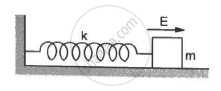Q 52 | Page 123

A block of mass containing a net positive charge q is placed on a smooth horizontal table which terminates in a vertical wall as shown in the figure. The distance of the block from the wall is d. A horizontal electric field E towards the right is switched on. Assuming elastic collisions (if any), find the time period of the resulting oscillatory motion. Is it a simple harmonic motion?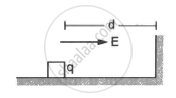Q 53 | Page 123

A uniform electric field of 10 N C−1 exists in the vertically downward direction. Find the increase in the electric potential as one goes up through a height of 50 cm.

Q 54 | Page 123

12 J of work has to be done against an existing electric field to take a charge of 0.01 C from A to B. How much is the potential difference  VB − VA

Q 55 | Page 123

Two equal charges, 2.0 × 10−7 C each, are held fixed at a separation of 20 cm. A third charge of equal magnitude is placed midway between the two charges. It is now moved to a point 20 cm from both the charges. How much work is done by the electric field during the process?

Q 56 | Page 123

An electric field of 20 NC−1 exists along the x-axis in space. Calculate the potential difference VB − VA where the points A and B are
(a) A = (0, 0); B = (4 m, 2m)
(b) A = (4 m, 2 m); B = (6 m, 5 m)
(c) A = (0, 0); B = (6 m, 5 m)
Do you find any relation between the answers of parts (a), (b) and (c)?

Q 57 | Page 123

Consider the situation of the previous problem. A charge of −2.0 × 10−4 C is moved from point A to point B. Find the change in electrical potential energy UB − UA for the cases (a), (b) and (c).

Q 58 | Page 123

An electric field  $\vec{E} = ( \vec{i} 20 + \vec{j} 30) {NC}^{- 1}$  exists in space. If the potential at the origin is taken to be zero, find the potential at (2 m, 2 m).

Q 59 | Page 123

An electric field  $\vec{E} = \vec{i}$  Ax exists in space, where A = 10 V m−2. Take the potential at (10 m, 20 m) to be zero. Find the potential at the origin.

Q 60 | Page 123

The electric potential existing in space is $\hspace{0.167em} V(x, y, z) = A(xy + yz + zx) .$ (a) Write the dimensional formula of A. (b) Find the expression for the electric field. (c) If A is 10 SI units, find the magnitude of the electric field at (1 m, 1 m, 1 m).

Q 61 | Page 123

Two charged particles, with equal charges of 2.0 × 10−5 C, are brought from infinity to within a separation of 10 cm. Find the increase in the electric potential energy during the process

Q 62 | Page 123

Some equipotential surface is shown in the figure. What can you say about the magnitude and the direction of the electric field?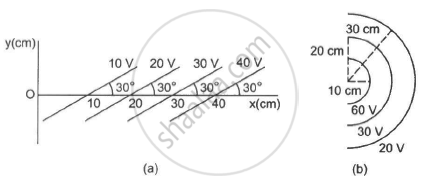Q 63 | Page 123

Consider a circular ring of radius r, uniformly charged with linear charge density λ. Find the electric potential at a point on the axis at a distance x from the centre of the ring. Using this expression for the potential, find the electric field at this point.

Q 64 | Page 123

An electric field of magnitude 1000 NC−1 is produced between two parallel plates with a separation of 2.0 cm, as shown in the figure. (a) What is the potential difference between the plates? (b) With what minimum speed should an electron be projected from the lower place in the direction of the field, so that it may reach the upper plate? (c) Suppose the electron is projected from the lower place with the speed calculated in part (b). The direction of projection makes an angle of 60° with the field. Find the maximum height reached by the electron.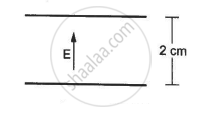Q 65 | Page 124

A uniform field of 2.0 NC−1 exists in space in the x-direction. (a) Taking the potential at the origin to be zero, write an expression for the potential at a general point (x, y, z). (b) At which point, the potential is 25 V? (c) If the potential at the origin is taken to be 100 V, what will be the expression for the potential at a general point? (d) What will be the potential at the origin if the potential at infinity is taken to be zero? Is it practical to choose the potential at infinity to be zero?

Q 66 | Page 124

How much work has to be done in assembling three charged particles at the vertices of an equilateral triangle, as shown in the figure?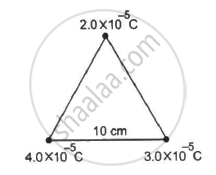Q 67 | Page 124

The kinetic energy of a charged particle decreases by 10 J as it moves from a point at potential 100 V to a point at potential 200 V. Find the charge on the particle.

Q 68 | Page 124

Two identical particles, each with a charge of 2.0 × 10−4 C and mass of 10 g, are kept at a separation of 10 cm and then released. What would be the speed of the particles when the separation becomes large?

Q 69 | Page 124

Two particles of masses 5.0 g each and opposite charges of +4.0 × 10−5 C and −4.0 × 10−5 C are released from rest with a separation of 1.0 m between them. Find the speeds of the particles when the separation is reduced to 50 cm.

Q 70 | Page 124

A sample of HCI gas is placed in an electric field of 2.5 × 104 NC−1. The dipole moment of each HCI molecule is 3.4 × 10−30 Cm. Find the maximum torque that can act on a molecule.

Q 71.1 | Page 124

Two particles A and B, of opposite charges 2.0 × 10−6 C and −2.0 × 10−6 C, are placed at a separation of 1.0 cm. Two particles A and B, of opposite charges 2.0 × 10−6 C and −2.0 × 10−6 C, are placed at a separation of 1.0 cm.

Q 71.2 | Page 124

Two particles and B, of opposite charges 2.0 × 10−6 C and −2.0 × 10−6 C, are placed at a separation of 1.0 cm. Calculate the electric field at a point on the axis of the dipole 1.0 cm away from the centre.

Q 71.3 | Page 124

Two particles A and B, of opposite charges 2.0 × 10−6 C and −2.0 × 10−6 C, are placed at a separation of 1.0 cm. Calculate the electric field at a point on the perpendicular bisector of the dipole and 1.0 m away from the centre.

Q 72 | Page 124

Three charges are arranged on the vertices of an equilateral triangle, as shown in the figure. Find the dipole moment of the combination.

Q 73 | Page 124

Find the magnitude of the electric field at the point P in the configuration shown in the figure for d >> a.

Q 74 | Page 124

Two particles, carrying charges −q and +q and and of mass m each, are fixed at the ends of a light rod of length a to form a dipole. The rod is clamped at an end and is placed in a uniform electric field E with the axis of the dipole along the electric field. The rod is slightly tilted and then released. Neglecting gravity, find the time period of small oscillations.

Q 75 | Page 124

Assume that each atom in a copper wire contributes one free electron. Estimate the number of free electrons in a copper wire of mass 6.4 g (take the atomic weight of copper to be 64 g mol−1).

## Chapter 7: Electric Field and Potential## HC Verma solutions for Class 12 Concepts of Physics Vol. 2 chapter 7 - Electric Field and Potential

HC Verma solutions for Class 12 Concepts of Physics Vol. 2 chapter 7 (Electric Field and Potential) include all questions with solution and detail explanation. This will clear students doubts about any question and improve application skills while preparing for board exams. The detailed, step-by-step solutions will help you understand the concepts better and clear your confusions, if any. Shaalaa.com has the CBSE Class 12 Concepts of Physics Vol. 2 solutions in a manner that help students grasp basic concepts better and faster.

Further, we at Shaalaa.com provide such solutions so that students can prepare for written exams. HC Verma textbook solutions can be a core help for self-study and acts as a perfect self-help guidance for students.

Concepts covered in Class 12 Concepts of Physics Vol. 2 chapter 7 Electric Field and Potential are Gauss’s Law, Physical Significance of Electric Field, Electric Field Due to a System of Charges, Charging by Induction, Electric Field Due to a Point Charge, Uniformly Charged Infinite Plane Sheet and Uniformly Charged Thin Spherical Shell (Field Inside and Outside), Applications of Gauss’s Law, Electric Flux, Dipole in a Uniform External Field, Electric Dipole, Electric Field Lines, Introduction of Electric Field, Continuous Charge Distribution, Superposition Principle of Forces, Forces Between Multiple Charges, Force Between Two Point Charges, Coulomb’s Law, Basic Properties of Electric Charge, Electric Charges.

Using HC Verma Class 12 solutions Electric Field and Potential exercise by students are an easy way to prepare for the exams, as they involve solutions arranged chapter-wise also page wise. The questions involved in HC Verma Solutions are important questions that can be asked in the final exam. Maximum students of CBSE Class 12 prefer HC Verma Textbook Solutions to score more in exam.

Get the free view of chapter 7 Electric Field and Potential Class 12 extra questions for Class 12 Concepts of Physics Vol. 2 and can use Shaalaa.com to keep it handy for your exam preparation